# Texas Go Math Grade 4 Lesson 2.4 Answer Key Relate Hundredths and Decimals

Refer to our Texas Go Math Grade 4 Answer Key Pdf to score good marks in the exams. Test yourself by practicing the problems from Texas Go Math Grade 4 Lesson 2.4 Answer Key Relate Hundredths and Decimals.

## Texas Go Math Grade 4 Lesson 2.4 Answer Key Relate Hundredths and Decimals

Essential Question

How can you record hundredths as fractions and decimals?
We know that,
The representation of the hundredths in the place-value chart is: 0.01
So,
The representation of the hundredths in the form of a fraction is: $$\frac{1}{100}$$
Hence, from the above,
We can conclude that
The representation of the hundredths in the form of a fraction is: $$\frac{1}{100}$$
The representation of the hundredths in the form of a decimal number is 0.01

Unlock the problem

In the 2008 Summer Olympic Games, the winning time in the men’s 100-meter butterfly race was only $$\frac{1}{100}$$ second faster than the second-place time. What decimal represents this fraction of a second?
• Circle the numbers you need to use.
It is given that
In the 2008 Summer Olympic Games, the winning time in the men’s 100-meter butterfly race was only $$\frac{1}{100}$$ second faster than the second-place time
Hence, from the above,
We can conclude that
The numbers we need to use are: 1 and 100

You can write hundredths as fractions or decimals.

One Way, Use a model and a place-value chart.
Fraction
Shade $$\frac{1}{100}$$ of the model.Write: 0.01

Decimal
Complete the place-value chart. $$\frac{1}{100}$$ is 1 hundredth.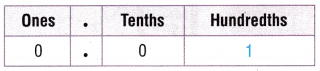Write: 0.01

Another Way Use a number line.
Label the number line with equivalent decimals. Locate the point $$\frac{1}{100}$$.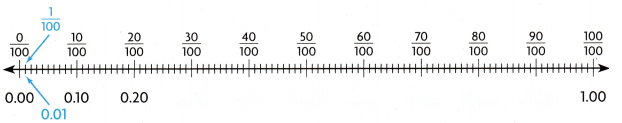Now,
From the above Number line,
We can observe that
0.01 names the same amount as $$\frac{1}{100}$$.
So,
The winning time was 0.01 seconds faster.

Math Talk

Mathematical Processes
Explain how the size of one-tenth is related to the size one hundredth.
We know that,
One-hundredth is the tenth of the one-tenth
Hence, from the above,
We can conclude that
One-hundredth = $$\frac{1}{10}$$ × One-tenth

Alicia Won her 400-meter freestyle race by 4$$\frac{25}{100}$$ seconds. How can you write this mixed number as a decimal?

Example Use a number line.
Label the number line with equivalent mixed numbers and decimals Locate the point 4$$\frac{25}{100}$$.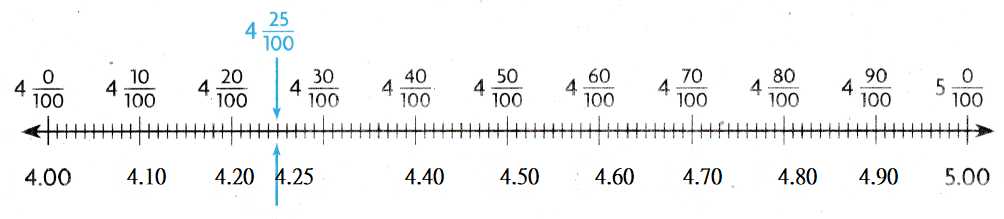Now,
From the above Number line,
We can observe that
4.25 names the same amount as 4$$\frac{25}{100}$$.
So,
Alicia won her race by 4.25 seconds.

Share and Show

Question 1.
Shade the model to show $$\frac{31}{100}$$
Write the amount as a decimal. ______________The given models are:Now,
To represent $$\frac{31}{100}$$ in a rectangle,
We will shade 31 boxes in a rectangle out of 100 boxes
Now,
To represent $$\frac{31}{100}$$ in the form of a decimal,
Write 31 after the decimal point
So,
The representation of $$\frac{31}{100}$$ in the given models is:Hence, from the above,
We can conclude that
The representation of $$\frac{31}{100}$$ in the form of a decimal is: 0.31

Write the fraction or mixed number and the decimal shown by the model.

Question 2.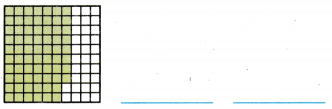The given model is: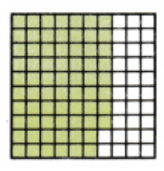Now,
From the above model,
We can observe that
The representation of the given model in the form of a fraction = (The number of shaded parts) ÷ (The total number of parts)
= $$\frac{68}{100}$$
Now,
The representation of the given model in the form of a decimal number is: 0.68
Hence, from the above,
We can conclude that
The representation of the given model in the form of a fraction is: $$\frac{68}{100}$$
The representation of the given model in the form of a decimal number is: 0.68

Question 3.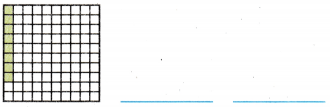The given model is: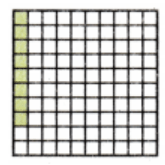Now,
From the above model,
We can observe that
The representation of the given model in the form of a fraction = (The number of shaded parts) ÷ (The total number of parts)
= $$\frac{8}{100}$$
Now,
The representation of the given model in the form of a decimal number is: 0.08
Hence, from the above,
We can conclude that
The representation of the given model in the form of a fraction is: $$\frac{8}{100}$$
The representation of the given model in the form of a decimal number is: 0.08

Question 4.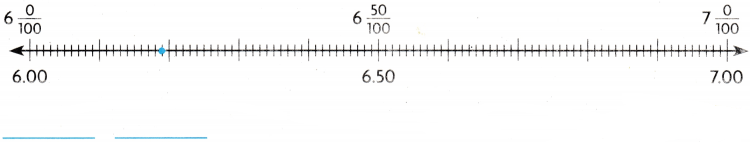The given model is: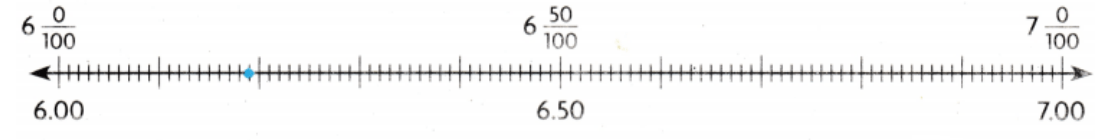Now,
The representation of the complete model is: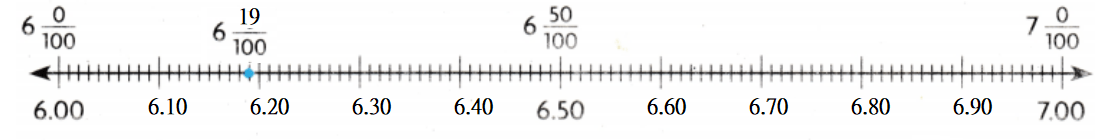Now,
From the above model,
We can observe that
The representation of the marked point in the form of a mixed number is: 6$$\frac{19}{100}$$
The representation of the marked point in the form of a decimal number is: 6.19
Hence, from the above,
We can conclude that
The representation of the marked point in the form of a mixed number is: 6$$\frac{19}{100}$$
The representation of the marked point in the form of a decimal number is: 6.19

Math Talk

Mathematical Processes
Are 0.5 and 0.50 equivalent? Explain.
The given decimal numbers are: 0.5 and 0.50
Now,
We know that,
If zero is placed on the right side as the last digit in the decimal number, then that zero don’t have any value
So,
0.5 = 0.50
Hence, from the above,
We can conclude that
0.5 and 0.50 are equivalent

Problem Solving

Question 5.
H.O.T Representations Shade the grids to show three different ways to represent $$\frac{16}{100}$$ using models.The given fraction is: $$\frac{16}{100}$$
Now,
To represent the given fraction in 3 different ways,
We have to write the equivalent fractions of $$\frac{16}{100}$$
So,
The equivalent fractions of the given fraction are:
$$\frac{16}{100}$$ = $$\frac{4}{25}$$ = $$\frac{8}{50}$$
So,
The representation of the above equivalent fractions in the models is: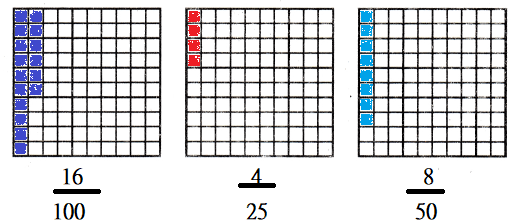Hence, from the above,
We can conclude that
The three ways to represent $$\frac{16}{100}$$ are:Question 6.
Write Math Explain how one whole, one-tenth, and one hundredth are related.
We know that,
“One-whole” is represented as 1
“One-tenth” is $$\frac{1}{10}$$ of 1
“One-hundredth” is $$\frac{1}{10}$$ of “One-tenth” and $$\frac{1}{100}$$ of 1
Hence, from the above,
We can conclude that
One-hundredth = $$\frac{1}{10}$$ of “One-tenth” = $$\frac{1}{100}$$ of 1

Sense or Nonsense?

Question 7.
H.O.T. Multi-Step The Memorial Library is 0.3 miles from the school. Whose statement makes sense? Whose statement is nonsense?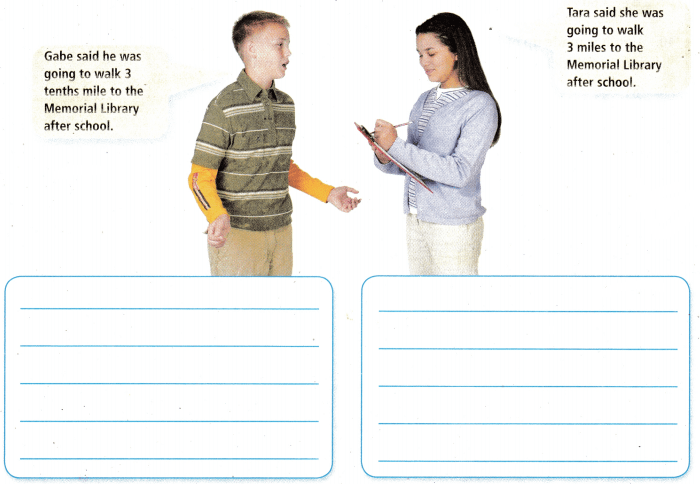It is given that
The Memorial Library is 0.3 miles from the school
Now,
The representation of 0.3 in the form of a fraction is: $$\frac{3}{10}$$
The representation of 0.3 in the word form is: Three tenths
Now,
According to the given information,
Gabe said he was going to walk three tenths miles
Tara said she was going to walk three miles
Hence, from the above,
We can conclude that
Tanya’s statement is nonsense

Question 8.
A house shaped like a ball has curved windows. The largest window is 2$$\frac{75}{100}$$ meters tall. What is 2$$\frac{75}{100}$$ written as a decimal?
(A) 0.275
(B) 2.175
(C)2.75
(D) 27.5
It is given that
A house shaped like a ball has curved windows. The largest window is 2$$\frac{75}{100}$$ meters tall
Now,
The given mixed number is: 2$$\frac{75}{100}$$
So,
2[llatex]\frac{75}{100}[/latex] = 2 + $$\frac{75}{100}$$
= 2 + 0.75
= 2.75
Hence, from the above,
We can conclude that
The representation of 2$$\frac{75}{100}$$ as a decimal is: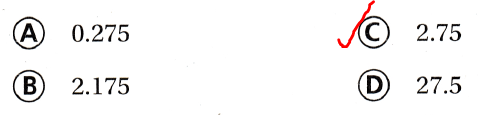Question 9.
Barry made the model below to show the distance he and his sister hiked while on vacation. Which decimal does the model show?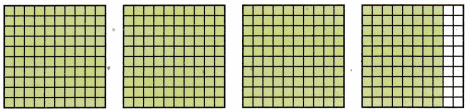(A) 4.80
(B) 3.80
(C) 4.08
(D) 3.08
It is given that
Barry made the model below to show the distance he and his sister hiked while on vacation
Now,
The given model is:Now,
From the above model,
We can observe that
The representation of the model in the form of a fraction = (The number of shaded parts) ÷ (The total number of parts)
= $$\frac{380}{100}[latex] = 3.80 Hence, from the above, We can conclude that The representation of the model in the form of a decimal number is:Question 10. A quilt is made up of 100 equal squares. There is a star on 35 of the squares. Which decimal represents the part of the quilt with stars? (A) 35 (B) 3.5 (C) 1.35 (D) 0.35 Answer: It is given that A quilt is made up of 100 equal squares. There is a star on 35 of the squares Now, The part of the quilt with stars = (The number of stars present) ÷ (The total number of squares) = [latex]\frac{35}{100}$$
= 0.35
Hence, from the above,
We can conclude that
The representation of the part of the quilt with the stars in the form of a decimal is:TEXAS Test Prep

Question 11.
Which are the fraction and decimal shown by the model?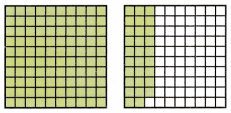(A) 12$$\frac{9}{10}$$; 12.9
(B) 1$$\frac{29}{100}$$; 1.29
(C) 1$$\frac{09}{100}$$; 1.09
(D) 2$$\frac{90}{100}$$; 2.90
The given model is:Now,
From the above model,
We can observe that
The representation of the above model in the form of a fraction = (The number of shaded parts) ÷ (The total number of parts)
= $$\frac{129}{100}$$
= 1$$\frac{29}{100}$$
= 1 + $$\frac{29}{100}$$
= 1 + 0.29
= 1.29
Hence, from the above,
We can conclude that
The representation of the fraction and decimal for the given model is:### Texas Go Math Grade 4 Lesson 2.4 Homework and Practice Answer Key

Relate Hundredths and Decimals

Question 1.
The fully shaded grid shows 1. Explain how these three models are related.
Problem Solving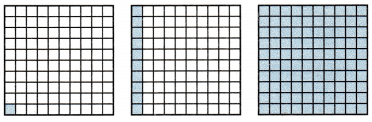It is given that
The fully shaded grid shows 1
Now,
The given model is:Now,
From the above model,
We can observe that
The first model is shaded only one-tenth of the second model
The second model is shaded only one-tenth of the third model
So,
The relationship between the models is:
1st model = $$\frac{1}{10}$$ of 2nd model = $$\frac{1}{100}$$ of 3rd model
Hence, from the above,
We can conclude that
The relationship between the models is:
1st model = $$\frac{1}{10}$$ of 2nd model = $$\frac{1}{100}$$ of 3rd model

Question 2.
Emma and Charles saw this sign along the highway.
Emma said, “It is seventy-five hundredths of a mile to Waterslide Park.”
Charles said, “It is seven and five-tenths miles to Waterslide Park.”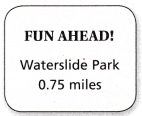Whose statement makes sense? whose statement is nonsense? Explain your reasoning.
It is given that
Emma said, “It is seventy-five hundredths of a mile to Waterslide Park.”
Charles said, “It is seven and five-tenths miles to Waterslide Park.”
Now,
The representation of seventy-five hundredths in the Standard form is: 0.75
The representation of seven and five-tenths in the Standard form is: 7.5
It is given that
The Waterslide park is: 0.75 miles
Hence, from the above,
We can conclude that
Emma’s statement makes sense
Charles’s statement makes not sense

Question 3.
Jake said he has $$\frac{8}{100}$$ of a dollar in his pocket. Margie said that the decimal for $$\frac{8}{100}$$ is 0.8. What error did she make? What is the decimal form for $$\frac{8}{100}$$?
It is given that
Jake said he has $$\frac{8}{100}$$ of a dollar in his pocket. Margie said that the decimal for $$\frac{8}{100}$$ is 0.8
Now,
The representation of $$\frac{8}{100}$$ in the decimal form is: 0.08
Now,
0.8 represents only one digit after the decimal point
But,
From the given fraction,
The digits after the decimal point must have 2
So,
The error made by Margie is:
The misinterprettion of digits after the decimal point
Hence, from the above,
We can conclude that
The error made by Margie is:
The misinterprettion of digits after the decimal point
The representation of $$\frac{8}{100}$$ in the decimal form is: 0.08

Lesson Check

Question 4.
What is the decimal for $$\frac{73}{100}$$?
(A) 7.3
(B) 0.73
(C) 73
(D) 100.73
The given fraction is: $$\frac{73}{100}$$
Now,
The representation of $$\frac{73}{100}$$ in the decimal form is: 0.73
Hence, from the above,
We can conclude that
The representation of $$\frac{73}{100}$$ in the decimal form is: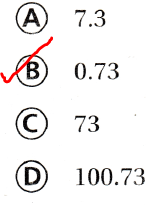Question 5.
A fully shaded grid shows 1. Which is the correct fraction or decimal for this model?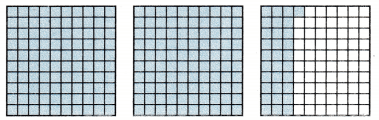(A) 231
(B) 2.31
(C) 2$$\frac{3}{100}$$
(D) $$\frac{231}{100}$$
It is given that
A fully shaded grid shows 1
Now,
The given model is:Now,
From the above model,
We can observe that
The representation of the given model in the form of a decimal number = 1 + 1 + $$\frac{31]{100}$$
= 2 + 0.31
= 2.31
= $$\frac{231}{100}$$
= 2$$\frac{31}{100}$$
Hence, from the above,
We can conclude that
The correct representation of the fraction and decimal for the given model is:Question 6.
Which fraction is marked with a dot on this number line?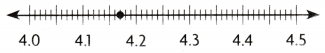(A) 4$$\frac{7}{10}$$
(B) 4$$\frac{7}{100}$$
(C) 4$$\frac{17}{100}$$
(D) 4$$\frac{17}{10}$$
The given model is:Now,
The representation of the complete model is:Now,
From the above model,
We can observe that
The dotted marked point is represented as: 4.17
So,
The representation of 4.17 in the form of a mixed number is: 4$$\frac{17}{100}$$
Hence, from the above,
We can conclude that
The representation of the dotted line on the number line in the form of a fraction is: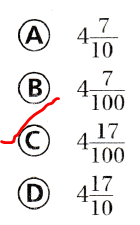Question 7.
Multi-Step A dollar is 100 cents. A quarter is 25 cents. What fraction of a dollar is a quarter?
(A) $$\frac{4}{100}$$
(B) $$\frac{100}{25}$$
(C) $$\frac{25}{10}$$
(D) $$\frac{25}{100}$$
The fraction of a dollar is a quarter = $$\frac{25]{100}$$